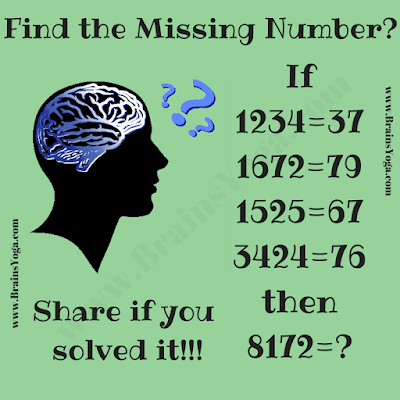Here is the time to test your logical skills. Here are some logical equations in this picture puzzle. These logical equations are incorrect Mathematically but these equations are correct logically with some pattern or series. Can you find this logical pattern that makes these equations correct and then find the missing number which will replace the question mark?Can you find the missing number?

Unknown said...

Ans: 99

Rajesh Kumar said...